Create a new printableFractions
Math Worksheets

Sample - Click above to make a new math worksheet (PDF).
 Name _____________________________Date ___________________
Percents
Write each decimal as a percent.
 1 0.6
 2 0.07
 3 0.77
 4 0.85
 5 0.52
 6 0.11
 7 0.44
 8 0.36
 9 0.08
 10 0.93

Write each fraction, decimal, or ratio as a percent.
 11 3:100
12.
 48100
 13 0.15
14.
 20100
 15 0.73
 16 39:100
17.
 91100
 18 0.06
 19 86:100
20.
 67100

Write each percent as a fraction in simplest form..
 21 80%
 22 55%
 23 1%
 24 67%
 25 16%
 26 41%
 27 39%
 28 22%
 29 93%
 30 5%

Write each percent as a ratio in simplest form..
 31 27%
 32 93%
 33 74%
 34 8%
 35 42%
 36 5%
 37 58%
 38 80%
 39 35%
 40 69%

 Key #2
 aKey #2
Find each missing number.
41.
 n = 21%
(n is a decimal)

42.
n%  =
 150

43.
17:n  =
 17100

44.
 7n
=  35%

45.
 31:n = 0.62

46.
n  =
 4350
(n is a decimal)
47.
 n100
=  7:100

48.
 n% = 29:50

49.
 n100
=  93:100

50.
 7:n = 0.7

Order each set from greatest to least.
51.
 47:50 35% 17:20

52.
 2750
89%
 83100
53.
 0.02 0.07 3:5

54.
0.11   6:25
 73100
55.
0.63
 625
60%
56.
 78% 0.28 27%

Order each set from least to greatest.
57.
1:10   0.01   89%   0.2
 750

 9100
58.
 77:100 39% 67:100 4:5 32% 0.59

59.
 720
0.21   30%
 43100
91%
 825
60.
0.45   47:50   0.16   21%   7:20
 325

Find 10% of each number. Round to the nearest hundredth.
 61 760
 62 7
 63 \$3.14
 64 13
 65 7.3
 66 5.03
 67 839
 68 54
 69 5.1
 70 7.06

 Key #2
 aKey #2
Find the percent of each number. Round your answer to the nearest tenth.
 71 35% of 10
 72 65% of 190
 73 85% of 140
 74 45% of 80
 75 60% of 20
 76 25% of 30

Find the percent of each number. Round your answer to the nearest tenth.
 77 75% of 150 = ______
 78 65% of 20 = ______
 79 30% of ______ = 18
 80 70% of ______ = 140
 81 25% of 180 = ______
 82 15% of 100 = ______

Complete.
 83 Write three decimals, three fractions, and three percents. Then order your set of numbers from least to greatest.
 84 Explain a method for converting any decimal number into a percent.

Complete.
85.
What Students Drank for Breakfast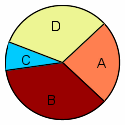A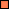Orange Juice  24% B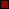Water  36% C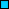Soda  8% D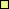Milk  32%

 a.  What fraction of the students drank soda for breakfast?b.  If the school has 120 students, how many students drank orange juice for breakfast?